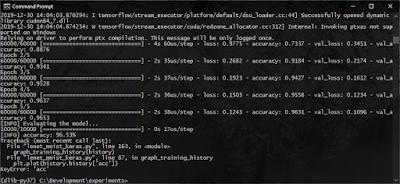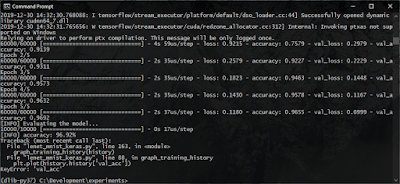# Fixing the KeyError: 'acc' and KeyError: 'val_acc' Errors in Keras 2.3.x

Have you been using the 'History' object returned by the fit() functions of Keras to graph or visualize the training history of your models? And have you been getting a 'KeyError' type error such as the following since recent Keras upgrade and wondering why?

```Traceback (most recent call last):
File "lenet_mnist_keras.py", line 163, in <module>
graph_training_history(history)
File "lenet_mnist_keras.py", line 87, in graph_training_history
plt.plot(history.history['acc'])
KeyError: 'acc'
```The KeyError: 'acc' when attempting to read the history object

```Traceback (most recent call last):
File "lenet_mnist_keras.py", line 163, in <module>
graph_training_history(history)
File "lenet_mnist_keras.py", line 88, in graph_training_history
plt.plot(history.history['val_acc'])
KeyError: 'val_acc'
```The KeyError: 'val_acc' when attempting to read the history object

Well, this is due to a breaking change introduced in Keras release 2.3.0.

According to the 2.3.0 Release Notes:
"Metrics and losses are now reported under the exact name specified by the user (e.g. if you pass metrics=['acc'], your metric will be reported under the string "acc", not "accuracy", and inversely metrics=['accuracy'] will be reported under the string "accuracy"."
You can read the official release notes here: https://github.com/keras-team/keras/releases/tag/2.3.0

What this means is that if you specify metrics=["accuracy"] in the model.compile(), then the history object will have the keys as 'accuracy' and 'val_accuracy'. While if you specify it as metrics=["acc"] then they will be reported with the keys 'acc' and 'val_acc'.

Therefore, to fix the error, you should just use one standard across your code.

You can either use 'acc',

```...

model.compile(loss="categorical_crossentropy",
optimizer=opt, metrics=["acc"])

...

plt.figure(1)

# summarize history for accuracy

plt.subplot(211)
plt.plot(history.history['acc'])
plt.plot(history.history['val_acc'])
plt.title('Model Accuracy')
plt.ylabel('Accuracy')
plt.xlabel('Epoch')
plt.legend(['Training', 'Validation'], loc='lower right')

# summarize history for loss

plt.subplot(212)
plt.plot(history.history['loss'])
plt.plot(history.history['val_loss'])
plt.title('Model Loss')
plt.ylabel('Loss')
plt.xlabel('Epoch')
plt.legend(['Training', 'Validation'], loc='upper right')

plt.tight_layout()

plt.show()
```

Or, use 'accuracy'.

```...

model.compile(loss="categorical_crossentropy",
optimizer=opt, metrics=["accuracy"])

...

plt.figure(1)

# summarize history for accuracy

plt.subplot(211)
plt.plot(history.history['accuracy'])
plt.plot(history.history['val_accuracy'])
plt.title('Model Accuracy')
plt.ylabel('Accuracy')
plt.xlabel('Epoch')
plt.legend(['Training', 'Validation'], loc='lower right')

# summarize history for loss

plt.subplot(212)
plt.plot(history.history['loss'])
plt.plot(history.history['val_loss'])
plt.title('Model Loss')
plt.ylabel('Loss')
plt.xlabel('Epoch')
plt.legend(['Training', 'Validation'], loc='upper right')

plt.tight_layout()

plt.show()
```Visualizing the model training history working after the 'fix'

Just remember to use the same key in both in the metrics=[...] and where you access the keys from the history object.